# Asymptotically-stable solution

(diff) ← Older revision | Latest revision (diff) | Newer revision → (diff)

A solution of a differential system that is stable according to Lyapunov (cf. Lyapunov stability) and that attracts all the other solutions with sufficiently close initial values. Thus, the solution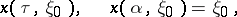of the system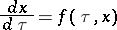(*)

with a right-hand side, given for all,, and which is such that solutions of (*) exist and are unique, will be an asymptotically-stable solution if, together with all its sufficiently close solutionsit is defined for all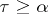and if for an arbitrarythere exists a,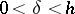, such thatimpliesfor alland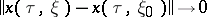as.

The concept of an asymptotically-stable solution was introduced by A.M. Lyapunov ; it, together with various special types of uniform asymptotic stability, is extensively used in the theory of stability , , .

How to Cite This Entry:
Asymptotically-stable solution. Encyclopedia of Mathematics. URL: http://encyclopediaofmath.org/index.php?title=Asymptotically-stable_solution&oldid=15407
This article was adapted from an original article by Yu.S. Bogdanov (originator), which appeared in Encyclopedia of Mathematics - ISBN 1402006098. See original article